# Consider the following steady, two-dimensional, incompressible velocity field:V=(u,v) = (-ax²)i + (2axy)j, where a is a constant. Calculate the pressure as a function of x and y.

Question-AnswerCategory: Fluid MechanicsConsider the following steady, two-dimensional, incompressible velocity field:V=(u,v) = (-ax²)i + (2axy)j, where a is a constant. Calculate the pressure as a function of x and y.

Consider the following steady, two-dimensional, incompressible velocity field:, where a is a constant. Calculate the pressure as a function of x and y.

Step: 1

Write the expression for steady, two dimensional, incompressible velocity field.Write the velocity components u and v.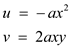Find the differentiation of velocity components.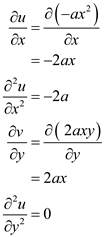Find the differentiation of velocity components with respect to time.Step: 2

Check the velocity field satisfies the two dimensional, incompressible continuity equation or not.
Write the two dimensional continuity equation.Substitutefor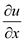and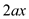forHence continuity equation satisfied.

Step: 3

Write the x-component of the two dimensional incompressible Navier-Stokes equation.Here,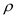is density,is gravity along x direction, andis dynamic viscosity.
Substitute 0 for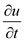, 0 for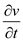, 0 for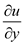forforfor u,for v,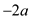for, 0 for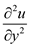, and 0 for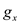Now, differentiate the above equation with respect to y.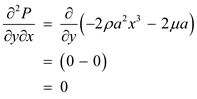Now, take the y-component of the two dimensional incompressible Navier-Stokes equation.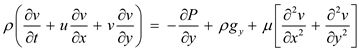Substitute 0 for, 0 for, 0 for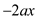for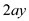for, 2ax for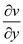for u,for v, 0 for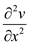, 0 for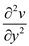, and 0 for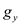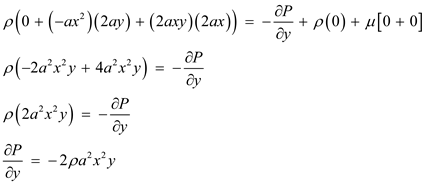Now, differentiate the above equation with respect to x.Since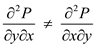Hence P is not a smooth function of x and y.
Therefore, the pressure as a function of x and y cannot be calculated.